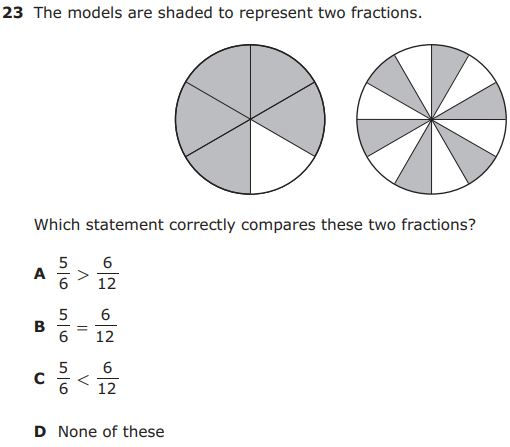top of page
Search

# 4th Grade Math Review: Day 3In yesterday's post we looked at rounding numbers and finding the connection between fractions and decimals. Today we finish the first reporting category (section) of the 3rd grade math curriculum, numerical representations and relationships, by looking at comparing fractions and finding fractions and decimals on a number line.

Comparing Fractions

Fractions up to this point in students' education have been compared with pictorial representations. Now 4th graders have to compare fractions by simply evaluating the numerators and denominators as well. The video below shows what this means for 4th graders.

With that reminder, try these practice problems from a released STAAR test.Fractions and Decimals on a Number Line

Students have learned about number lines for many years now. In the 4th grade, they apply that knowledge to placing both fractions and decimals on a number line as distances from zero. Here's a video that breaks down the concept.

With that in mind, try this problem out to test your skill.